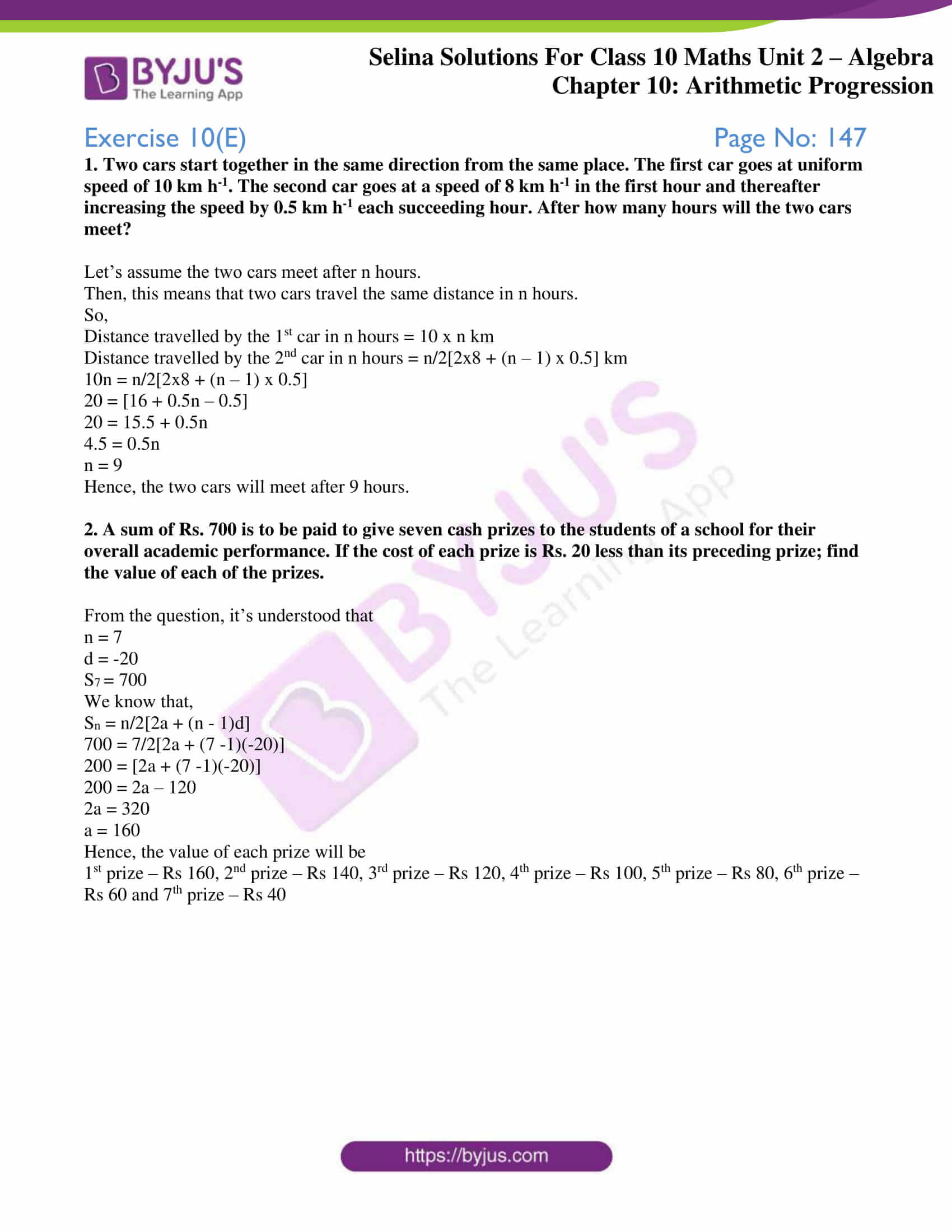# Selina Solutions Concise Maths Class 10 Chapter 10 Arithmetic Progression Exercise 10(E)

Exercise 10(E) contains word problems based on real-time situations. Students willing to have a good grip over solving problems of A.P can utilise the Selina Solutions for Class 10 Maths. All the solutions are developed by experts at BYJU’S, according to the latest ICSE marking schemes. For the solutions of this exercise, students can access the Selina Solutions Concise Maths for Class 10 Chapter 10 Arithmetic Progression Exercise 10(E), PDF in the links given below.

## Selina Solutions Concise Maths Class 10 Chapter 10 Arithmetic Progression Exercise 10(E) Download PDF### Access Selina Solutions Concise Maths Class 10 Chapter 10 Arithmetic Progression Exercise 10(E)

#### Exercise 10(E) Page No: 147

1. Two cars start together in the same direction from the same place. The first car goes at uniform speed of 10 km h-1. The second car goes at a speed of 8 km h-1 in the first hour and thereafter increasing the speed by 0.5 km h-1 each succeeding hour. After how many hours will the two cars meet?

Let’s assume the two cars meet after n hours.

Then, this means that two cars travel the same distance in n hours.

So,

Distance travelled by the 1st car in n hours = 10 x n km

Distance travelled by the 2nd car in n hours = n/2[2×8 + (n – 1) x 0.5] km

10n = n/2[2×8 + (n – 1) x 0.5]

20 = [16 + 0.5n – 0.5]

20 = 15.5 + 0.5n

4.5 = 0.5n

n = 9

Hence, the two cars will meet after 9 hours.

2. A sum of Rs. 700 is to be paid to give seven cash prizes to the students of a school for their overall academic performance. If the cost of each prize is Rs. 20 less than its preceding prize; find the value of each of the prizes.

From the question, it’s understood that

n = 7

d = -20

S7 = 700

We know that,

Sn = n/2[2a + (n – 1)d]

700 = 7/2[2a + (7 -1)(-20)]

200 = [2a + (7 -1)(-20)]

200 = 2a – 120

2a = 320

a = 160

Hence, the value of each prize will be

1st prize – Rs 160, 2nd prize – Rs 140, 3rd prize – Rs 120, 4th prize – Rs 100, 5th prize – Rs 80, 6th prize – Rs 60 and 7th prize – Rs 40

### Access other exercises of Selina Solutions Concise Maths Class 10 Chapter 10 Arithmetic Progression

Exercise 10(A) Solutions

Exercise 10(B) Solutions

Exercise 10(C) Solutions

Exercise 10(D) Solutions

Exercise 10(F) Solutions